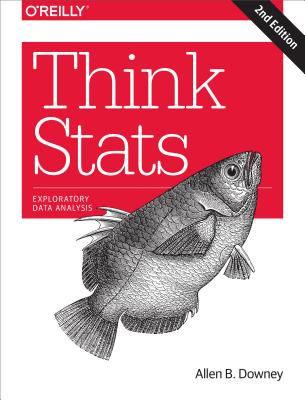### Think STATS: Exploratory Data Analysis### Huge savings for students

Each student receives a 50% discount off of most books in the HSG Book Store. During class, please ask the instructor about purchase details.
 List Price: \$34.99 Price: \$17.50 You Save: \$17.50
9

If you know how to program, you have the skills to turn data into knowledge, using tools of probability and statistics. This concise introduction shows you how to perform statistical analysis computationally, rather than mathematically, with programs written in Python.

By working with a single case study throughout this thoroughly revised book, you'll learn the entire process of exploratory data analysis--from collecting data and generating statistics to identifying patterns and testing hypotheses. You'll explore distributions, rules of probability, visualization, and many other tools and concepts.

New chapters on regression, time series analysis, survival analysis, and analytic methods will enrich your discoveries.

• Develop an understanding of probability and statistics by writing and testing code
• Run experiments to test statistical behavior, such as generating samples from several distributions
• Use simulations to understand concepts that are hard to grasp mathematically
• Import data from most sources with Python, rather than rely on data that's cleaned and formatted for statistics tools